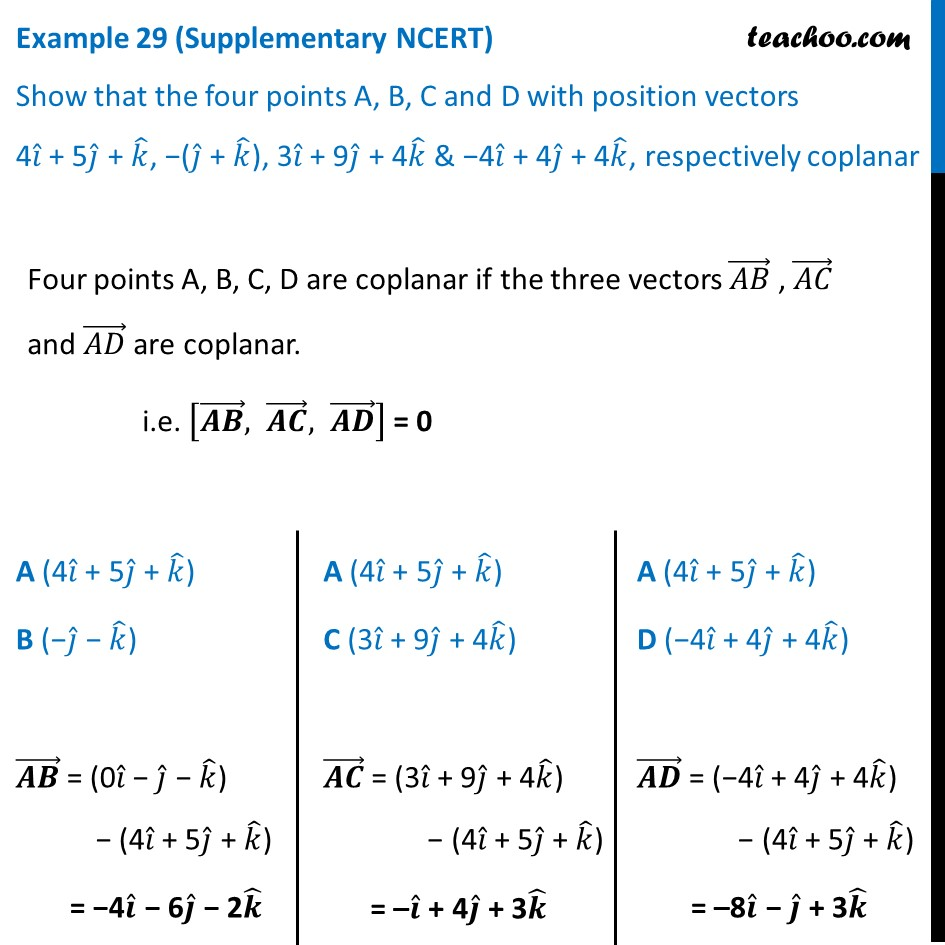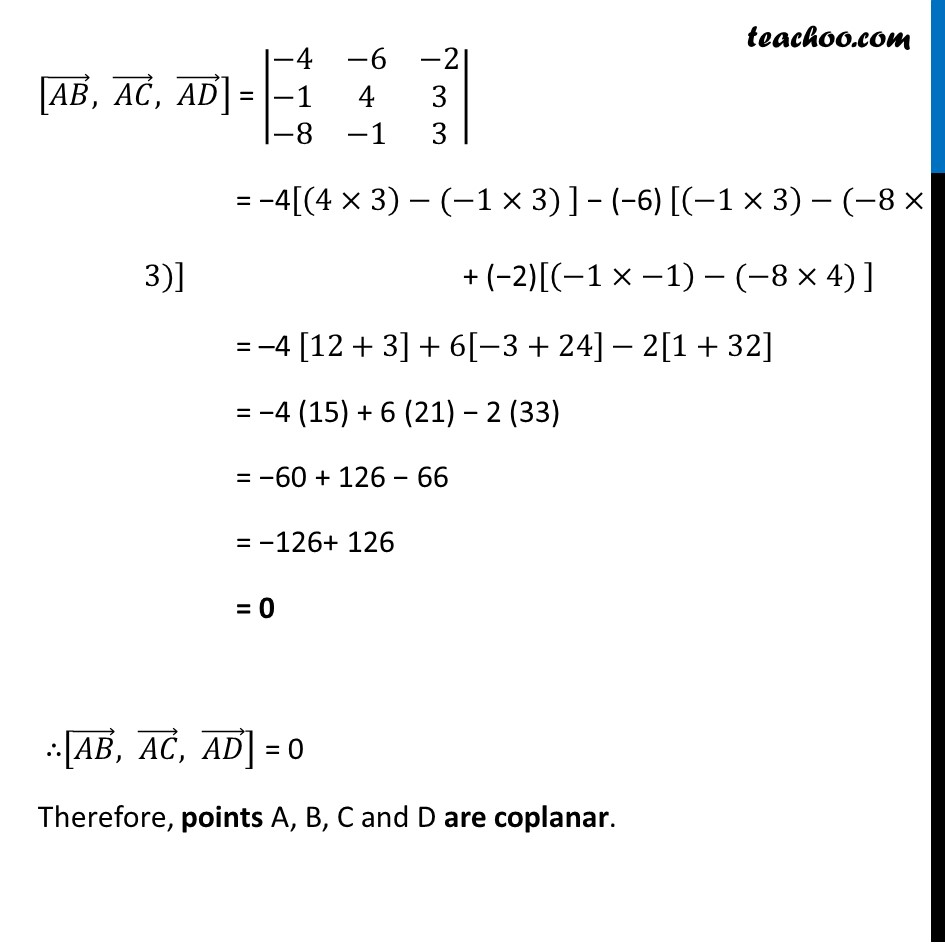Examples

Chapter 10 Class 12 Vector Algebra
Serial order wiseLearn in your speed, with individual attention - Teachoo Maths 1-on-1 Class

### Transcript

Example 29 (Supplementary NCERT) Show that the four points A, B, C and D with position vectors 4𝑖 ̂ + 5𝑗 ̂ + 𝑘 ̂, −(𝑗 ̂ + 𝑘 ̂), 3𝑖 ̂ + 9𝑗 ̂ + 4𝑘 ̂ & −4𝑖 ̂ + 4𝑗 ̂ + 4𝑘 ̂, respectively coplanar Four points A, B, C, D are coplanar if the three vectors (𝐴𝐵) ⃗ , (𝐴𝐶) ⃗ and (𝐴𝐷) ⃗ are coplanar. i.e. [(𝑨𝑩) ⃗, (𝑨𝑪) ⃗, (𝑨𝑫) ⃗ ] = 0 A (4𝑖 ̂ + 5𝑗 ̂ + 𝑘 ̂) B (−𝑗 ̂ − 𝑘 ̂) (𝑨𝑩) ⃗ = (0𝑖 ̂ − 𝑗 ̂ − 𝑘 ̂) − (4𝑖 ̂ + 5𝑗 ̂ + 𝑘 ̂) = −4𝒊 ̂ − 6𝒋 ̂ − 2𝒌 ̂ A (4𝑖 ̂ + 5𝑗 ̂ + 𝑘 ̂) C (3𝑖 ̂ + 9𝑗 ̂ + 4𝑘 ̂) (𝑨𝑪) ⃗ = (3𝑖 ̂ + 9𝑗 ̂ + 4𝑘 ̂) − (4𝑖 ̂ + 5𝑗 ̂ + 𝑘 ̂) = –𝒊 ̂ + 4𝒋 ̂ + 3𝒌 ̂ A (4𝑖 ̂ + 5𝑗 ̂ + 𝑘 ̂) D (−4𝑖 ̂ + 4𝑗 ̂ + 4𝑘 ̂) (𝑨𝑫) ⃗ = (−4𝑖 ̂ + 4𝑗 ̂ + 4𝑘 ̂) − (4𝑖 ̂ + 5𝑗 ̂ + 𝑘 ̂) = –8𝒊 ̂ − 𝒋 ̂ + 3𝒌 ̂ [(𝐴𝐵) ⃗, (𝐴𝐶) ⃗, (𝐴𝐷) ⃗ ] = |■8(−4&−6&−2@−1&4&3@−8&−1&3)| = −4[(4×3)−(−1×3) ] − (−6) [(−1×3)−(−8×3)] + (−2)[(−1×−1)−(−8×4) ] = –4 [12+3]+6[−3+24]−2[1+32] = −4 (15) + 6 (21) − 2 (33) = −60 + 126 − 66 = −126+ 126 = 0 ∴[(𝐴𝐵) ⃗, (𝐴𝐶) ⃗, (𝐴𝐷) ⃗ ] = 0 Therefore, points A, B, C and D are coplanar.